Examples

Chapter 6 Class 10 Triangles
Serial order wise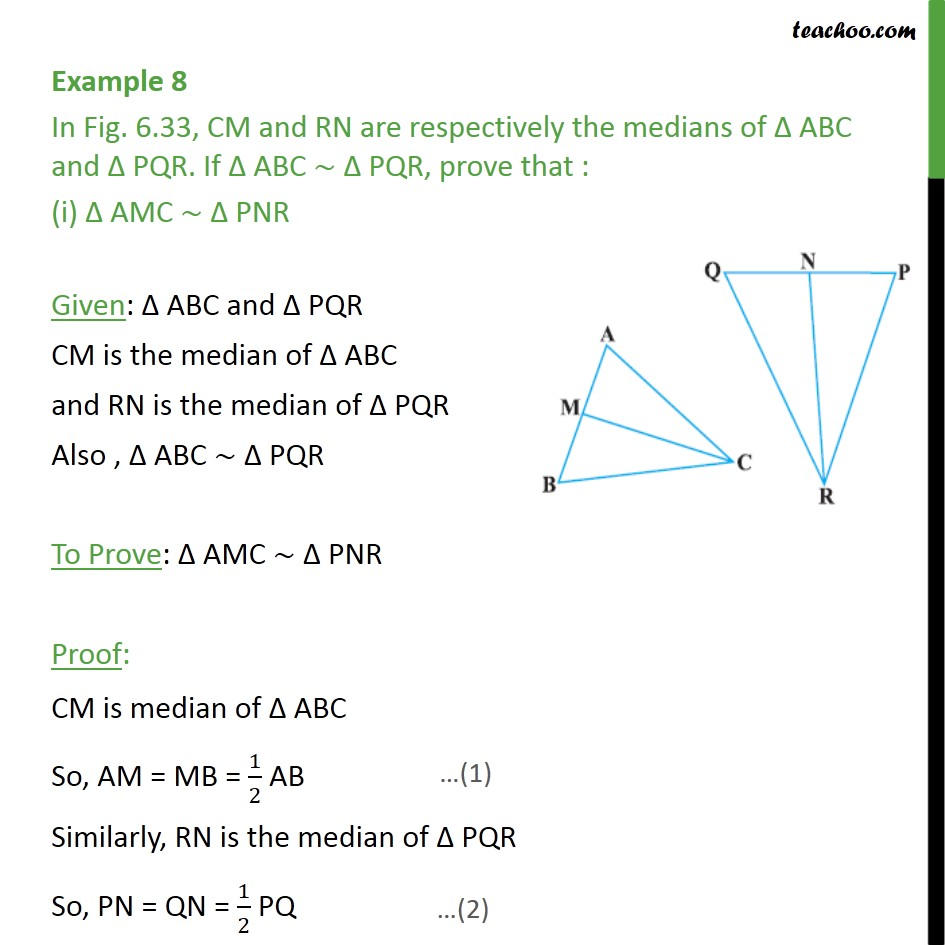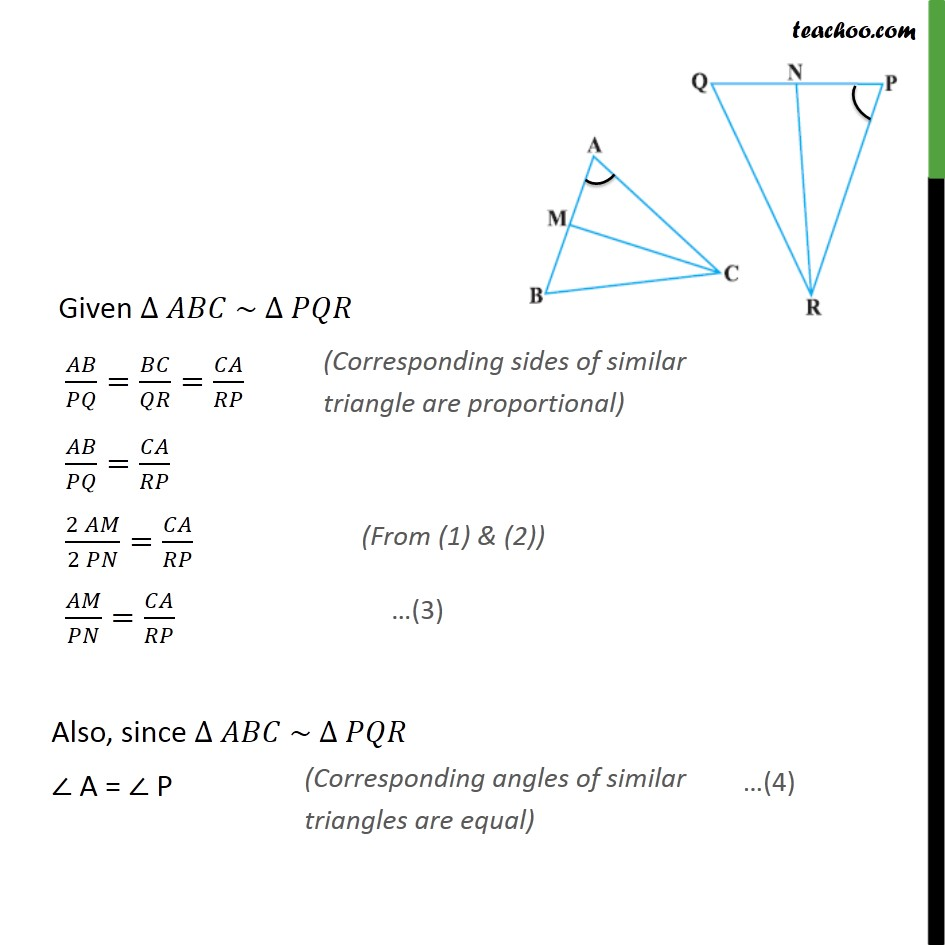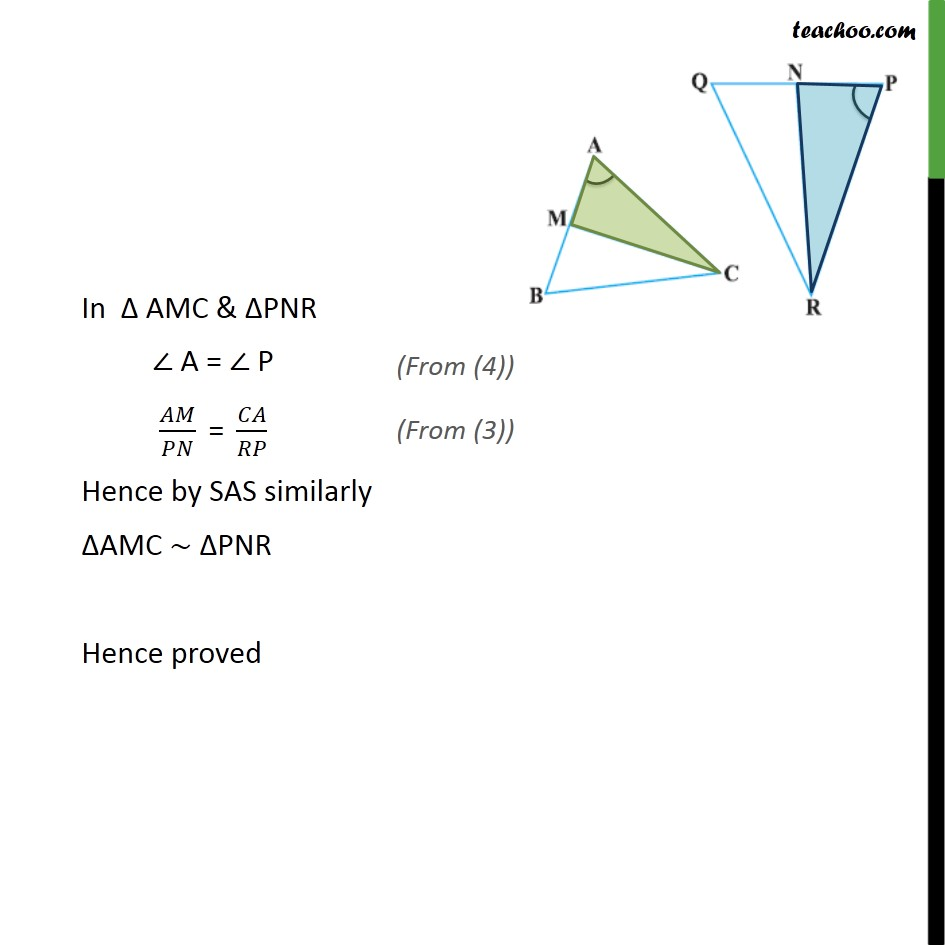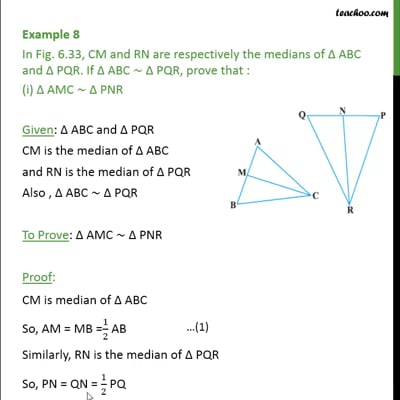This video is only available for Teachoo black users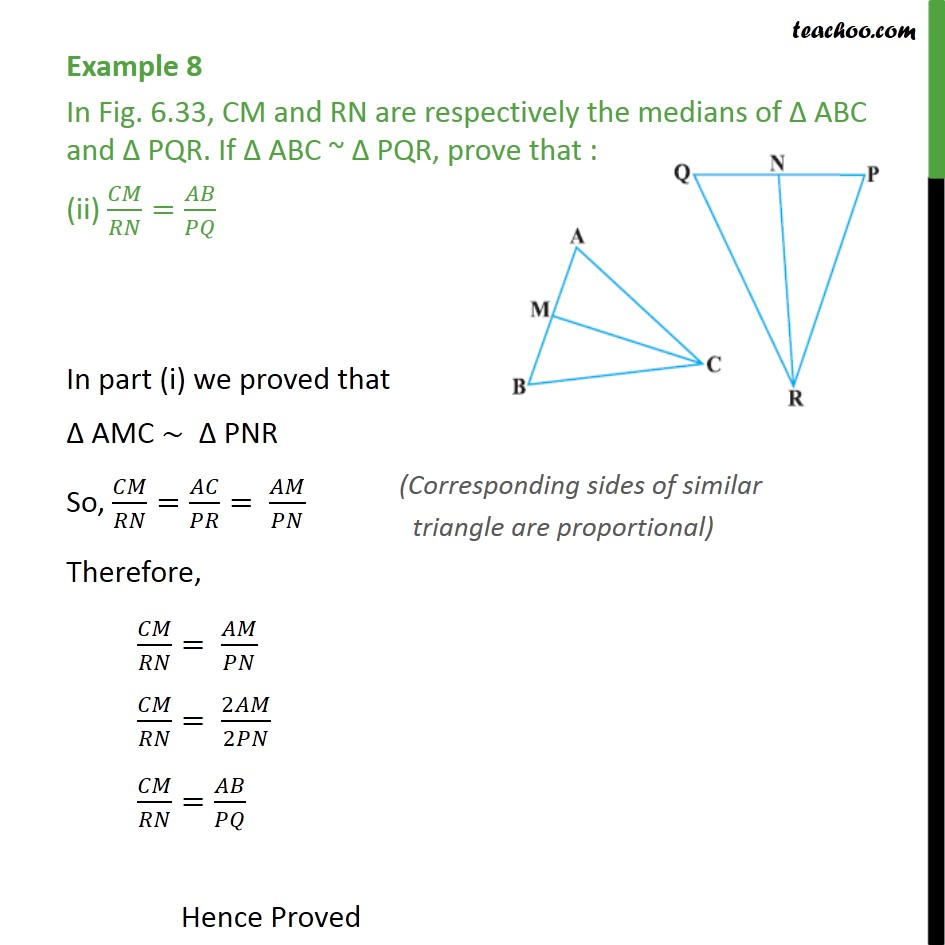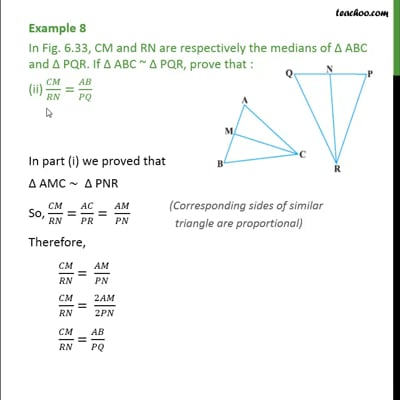This video is only available for Teachoo black users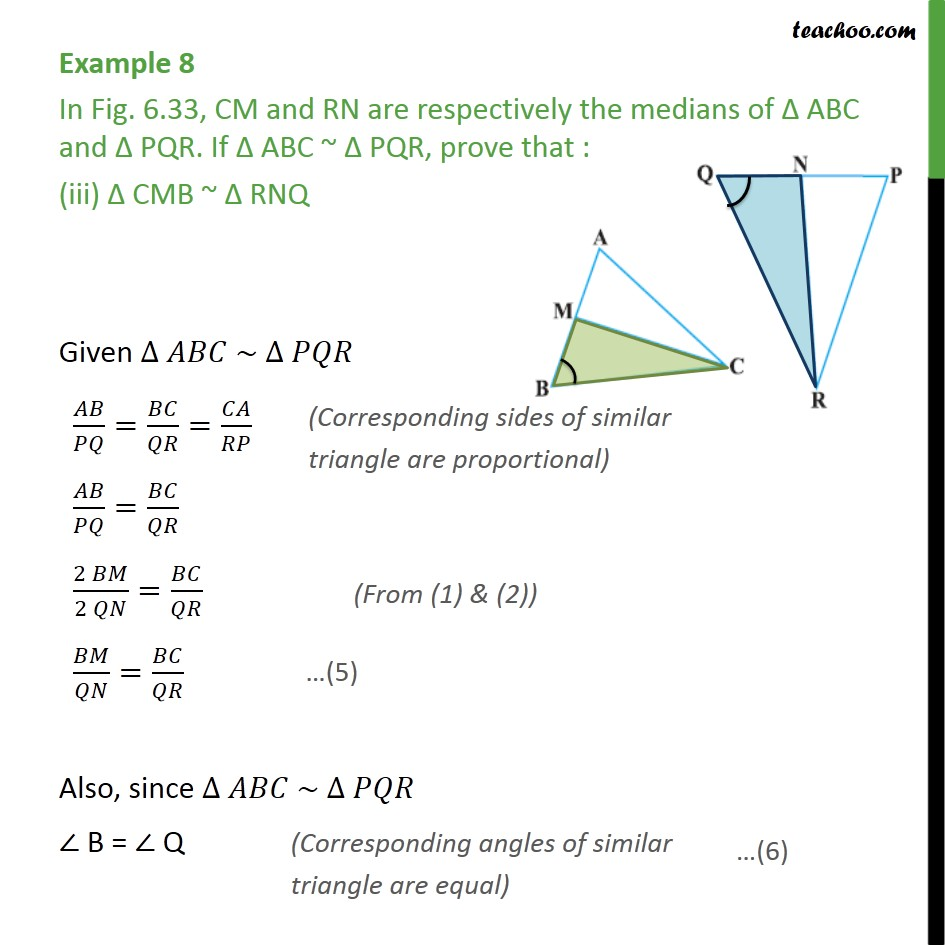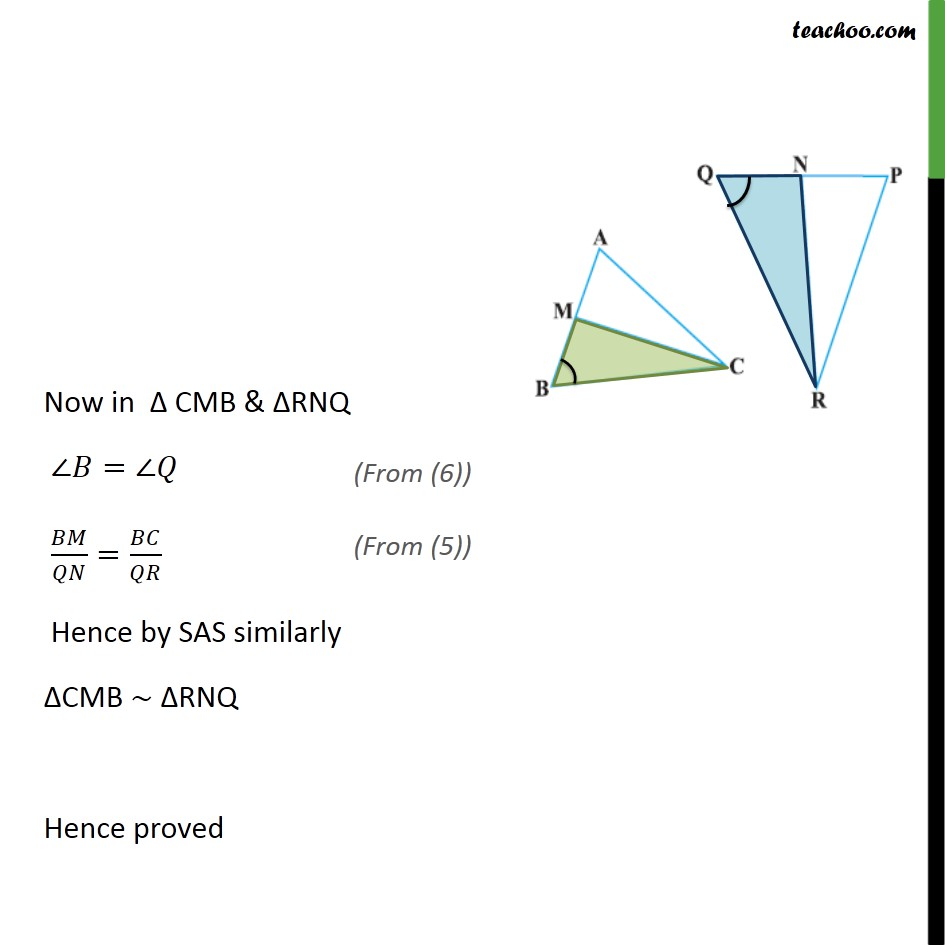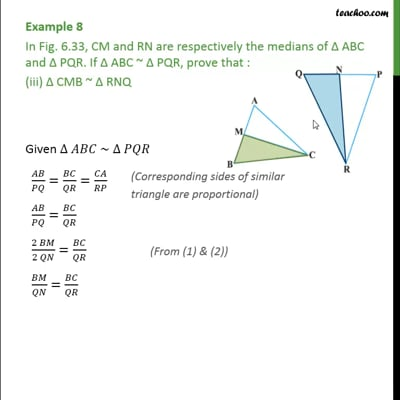This video is only available for Teachoo black users

Introducing your new favourite teacher - Teachoo Black, at only ₹83 per month

### Transcript

Example 8 In Fig. 6.33, CM and RN are respectively the medians of Δ ABC and Δ PQR. If Δ ABC ~ Δ PQR, prove that : (i) Δ AMC ~ Δ PNR Given: Δ ABC and Δ PQR CM is the median of Δ ABC and RN is the median of Δ PQR Also , Δ ABC ~ Δ PQR To Prove: Δ AMC ~ Δ PNR Proof: CM is median of Δ ABC So, AM = MB = 1/2 AB Similarly, RN is the median of Δ PQR So, PN = QN = 1/2 PQ Given ∆ 𝐴𝐵𝐶 ~ ∆ 𝑃𝑄𝑅 𝐴𝐵/𝑃𝑄=𝐵𝐶/𝑄𝑅=𝐶𝐴/𝑅𝑃 𝐴𝐵/𝑃𝑄=𝐶𝐴/𝑅𝑃 (2 𝐴𝑀)/(2 𝑃𝑁)=𝐶𝐴/𝑅𝑃 𝐴𝑀/𝑃𝑁=𝐶𝐴/𝑅𝑃 Also, since ∆ 𝐴𝐵𝐶 ~ ∆ 𝑃𝑄𝑅 ∠ A = ∠ P In Δ AMC & ΔPNR ∠ A = ∠ P 𝐴𝑀/𝑃𝑁 " = " 𝐶𝐴/𝑅𝑃 Hence by SAS similarly ΔAMC ∼ ΔPNR Hence proved Example 8 In Fig. 6.33, CM and RN are respectively the medians of Δ ABC and Δ PQR. If Δ ABC ~ Δ PQR, prove that : (ii) 𝐶𝑀/𝑅𝑁=𝐴𝐵/𝑃𝑄 In part (i) we proved that Δ AMC ~ Δ PNR So, 𝐶𝑀/𝑅𝑁=𝐴𝐶/𝑃𝑅= 𝐴𝑀/𝑃𝑁 Therefore, 𝐶𝑀/𝑅𝑁= 𝐴𝑀/𝑃𝑁 𝐶𝑀/𝑅𝑁= 2𝐴𝑀/2𝑃𝑁 𝐶𝑀/𝑅𝑁=𝐴𝐵/𝑃𝑄 Hence Proved Example 8 In Fig. 6.33, CM and RN are respectively the medians of Δ ABC and Δ PQR. If Δ ABC ~ Δ PQR, prove that : (iii) Δ CMB ~ Δ RNQ Given ∆ 𝐴𝐵𝐶 ~ ∆ 𝑃𝑄𝑅 𝐴𝐵/𝑃𝑄=𝐵𝐶/𝑄𝑅=𝐶𝐴/𝑅𝑃 𝐴𝐵/𝑃𝑄=𝐵𝐶/𝑄𝑅 (2 𝐵𝑀)/(2 𝑄𝑁)=𝐵𝐶/𝑄𝑅 𝐵𝑀/𝑄𝑁=𝐵𝐶/𝑄𝑅 Also, since ∆ 𝐴𝐵𝐶 ~ ∆ 𝑃𝑄𝑅 ∠ B = ∠ Q Now in Δ CMB & ΔRNQ ∠𝐵=∠𝑄 𝐵𝑀/𝑄𝑁=𝐵𝐶/𝑄𝑅 Hence by SAS similarly ΔCMB ∼ ΔRNQ Hence proved